This is year 1 Numeracy

This is year 1 Numeracy and category is

Filters

### Properties of 2D shapes

Students recognise and identify the names and properties of common 2D shapes.

Age: 5 - 6
1
2
3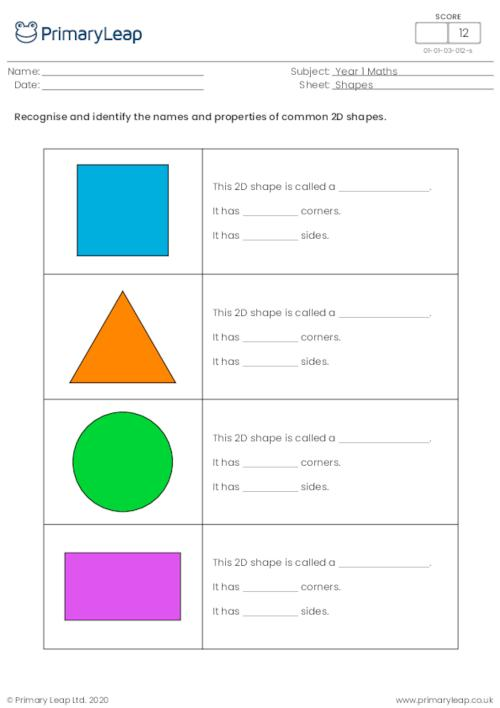### Naming 3D shapes

Students name and colour the 3D shapes.

Age: 5 - 6
1
2
3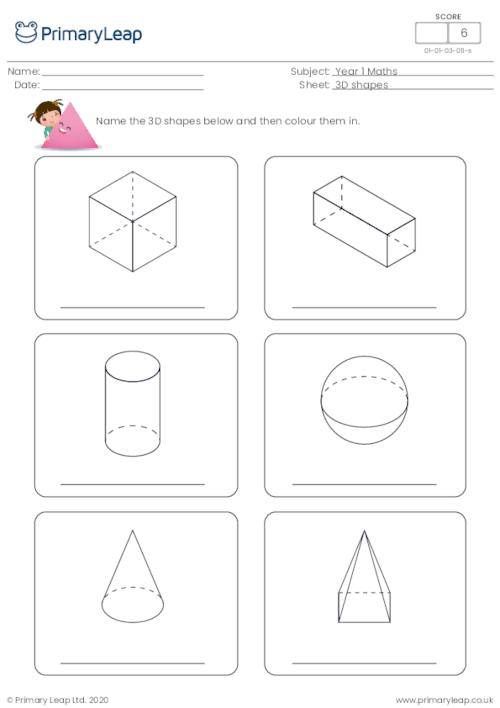### Read and match 3D shapes

Students read and match the 3D shapes by drawing a line.

Age: 5 - 6
1
2
3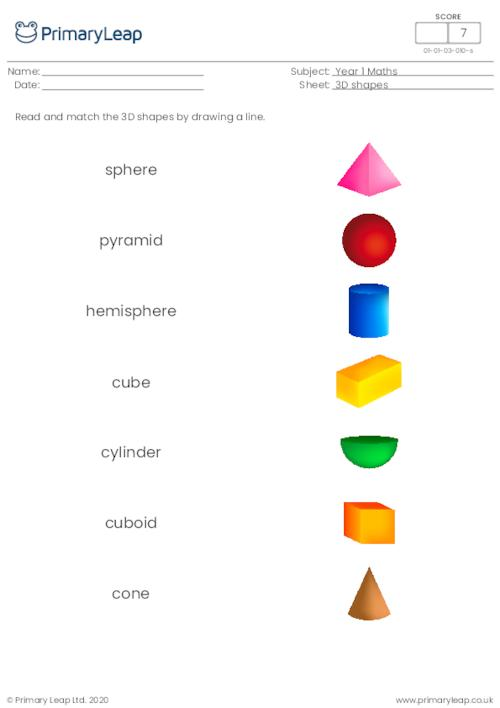### Ordering objects by length

Children write 1, 2 and 3 in the boxes to show the order of the objects from shortest to longest.

Age: 5 - 6
1
2
3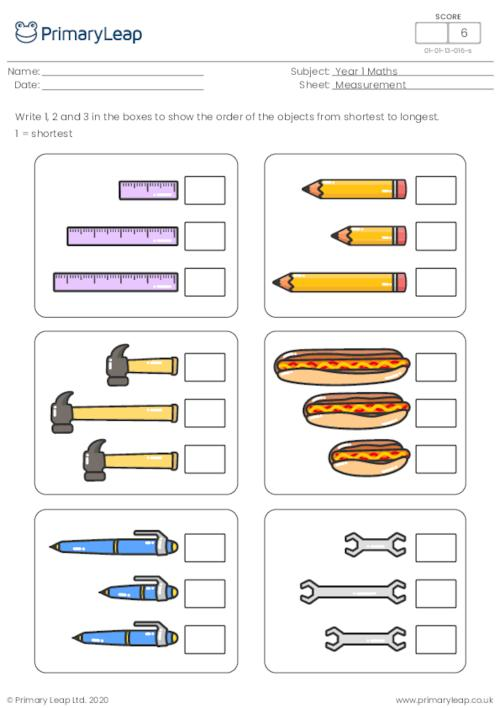### Measuring objects

Children look at the rulers and write down the measurement of each object.

Age: 5 - 6
1
2
3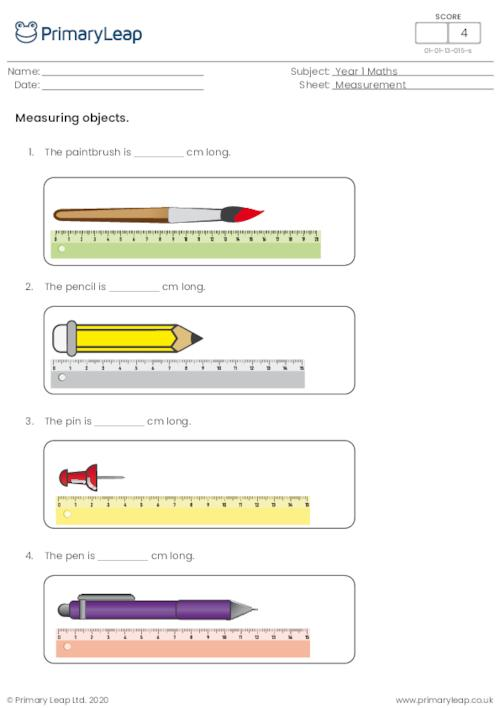### Which is the tallest?

Measurement - Children colour the tallest object in each group.

Age: 5 - 6
1
2
3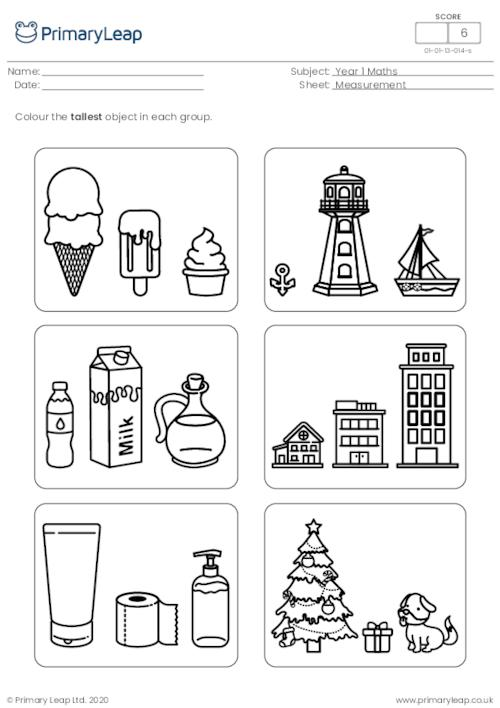### Smallest size

Measurement - Children colour the smallest object in each group.

Age: 5 - 6
1
2
3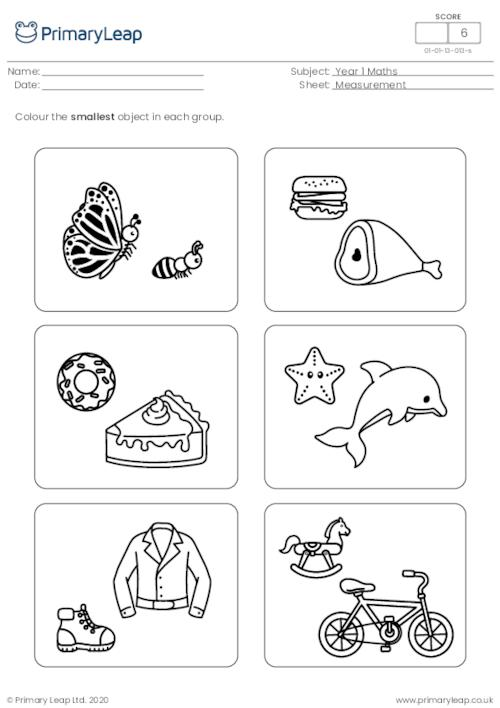### Longest length

Measurement - Children colour the longest object in each group.

Age: 5 - 6
1
2
3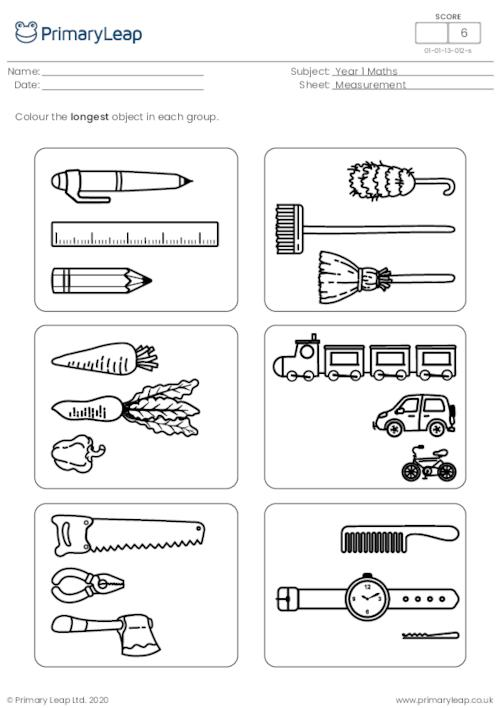### How tall?

Children colour the correct number of blocks to show the height of each object and then write the number in the box.

Age: 5 - 6
1
2
3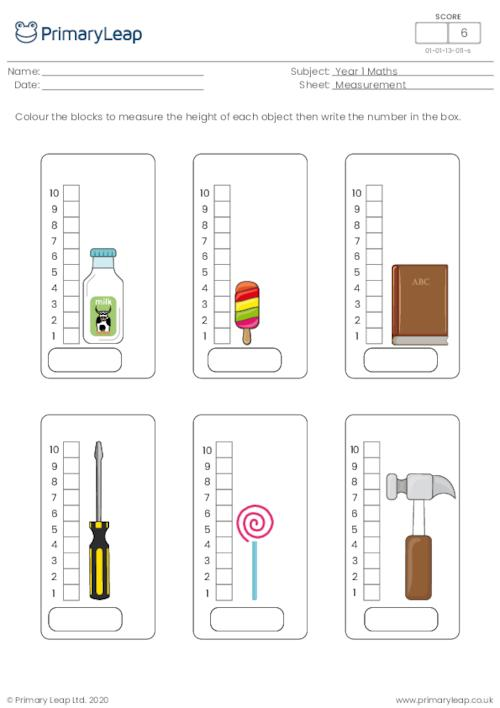### Ordering by length

Children number the objects from shortest to tallest/longest.

Age: 5 - 6
1
2
3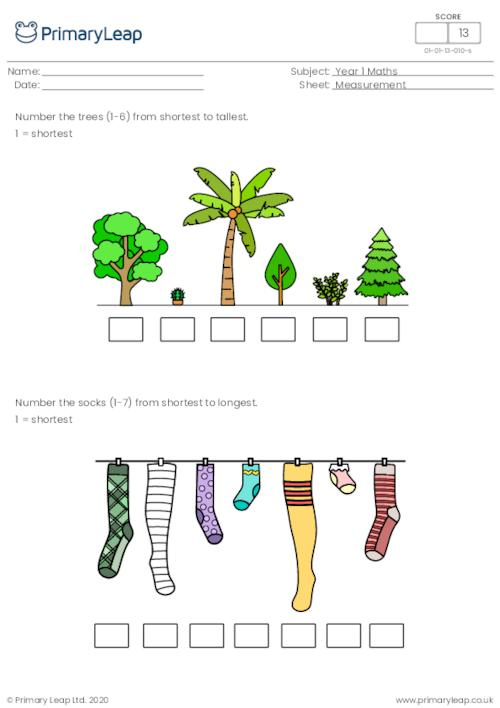### Doubling numbers with shapes

This maths worksheet asks students to double the number of shapes and draw the correct number in each box.

Age: 5 - 6
1
2
3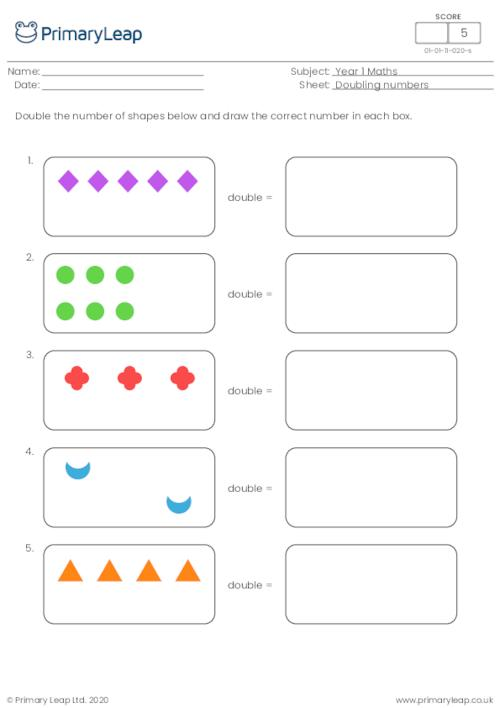### Doubling numbers

This maths worksheet asks students to double the number of sweets and draw the correct number in the box.

Age: 5 - 6
1
2
3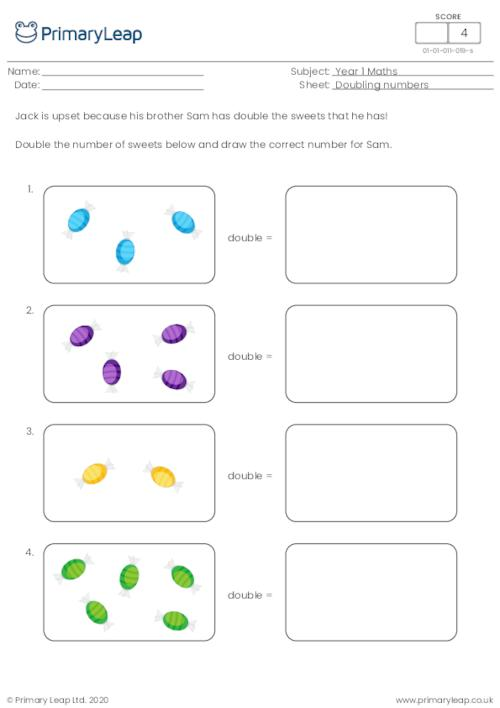### Colour half (shapes)

Children colour half the number of shapes.

Age: 5 - 6
1
2
3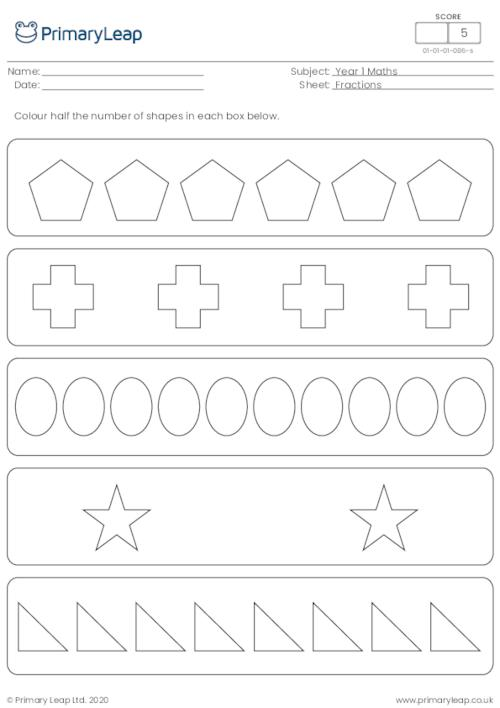### Colour half (sea animals)

Children colour half the number of sea animals.

Age: 5 - 6
1
2
3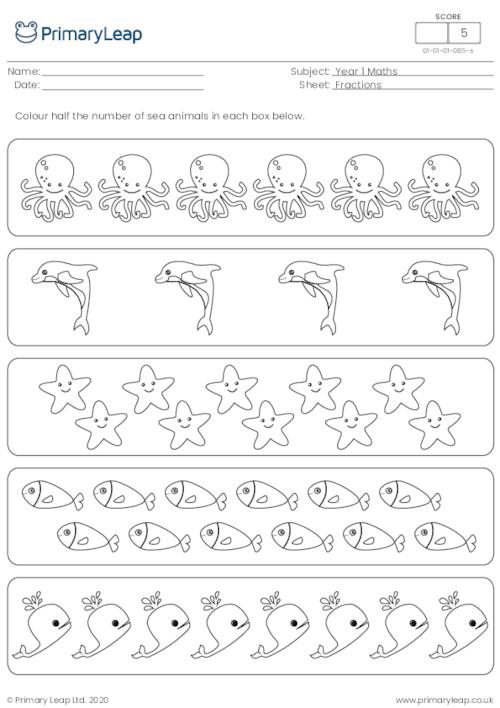### Colour half of each shape

Children colour half of each shape. Use a different colour to make your worksheet more colourful!

Age: 5 - 6
1
2
3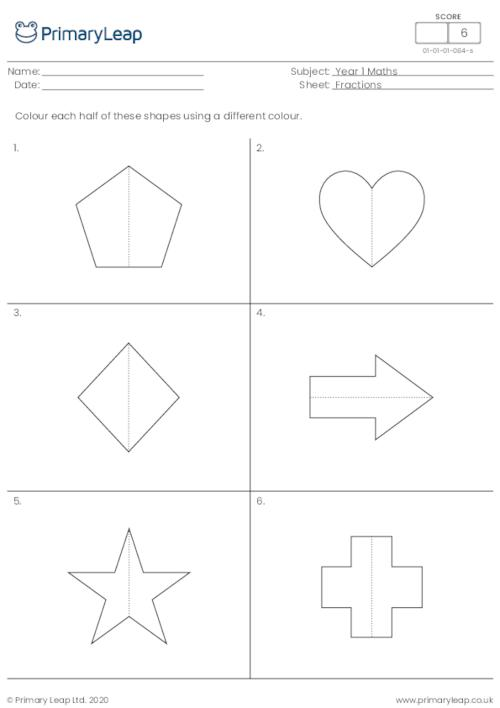### Colour a quarter of each shape

Children colour a quarter of each shape. Use a different colour to make your worksheet more colourful!

Age: 5 - 6
1
2
3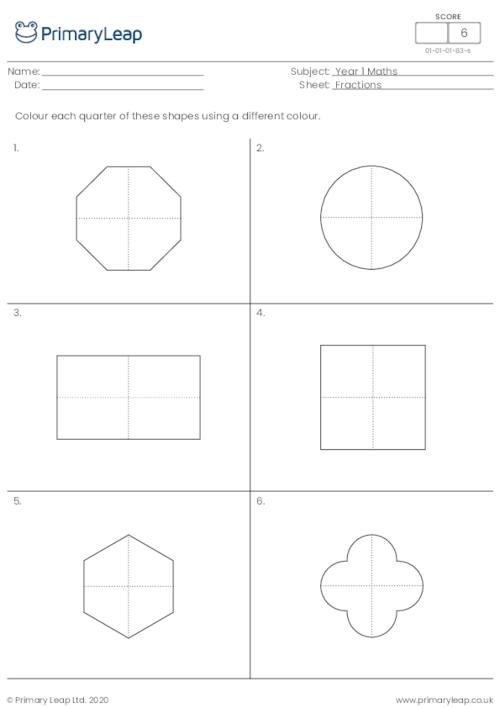### Write the fraction

Children write the correct fraction of the shaded part of each shape.

Age: 5 - 6
1
2
3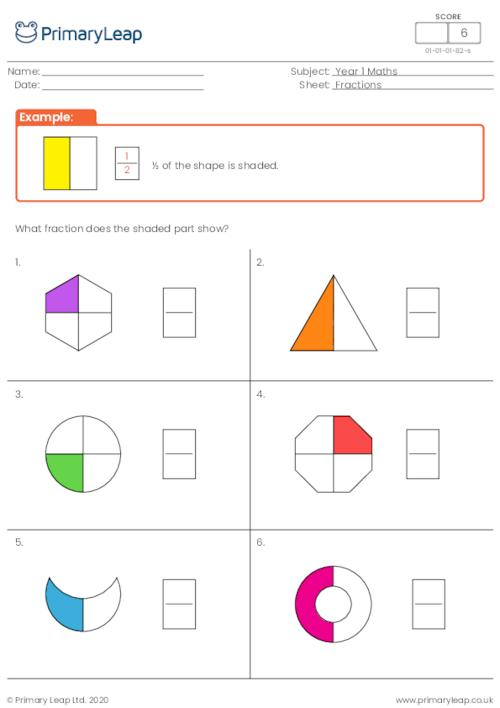### Finding a quarter

Children find a quarter of the number of insects in each box by drawing a circle.

Age: 5 - 6
1
2
3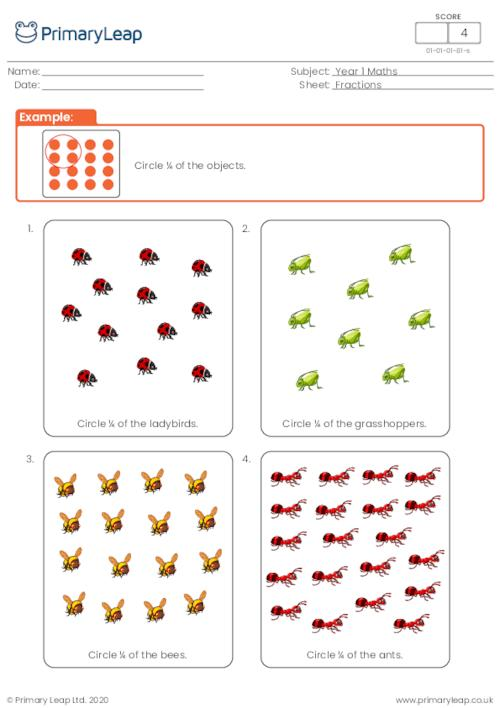### Finding half

Children find half the amount of fruit in each box by drawing a circle.

Age: 5 - 6
1
2
3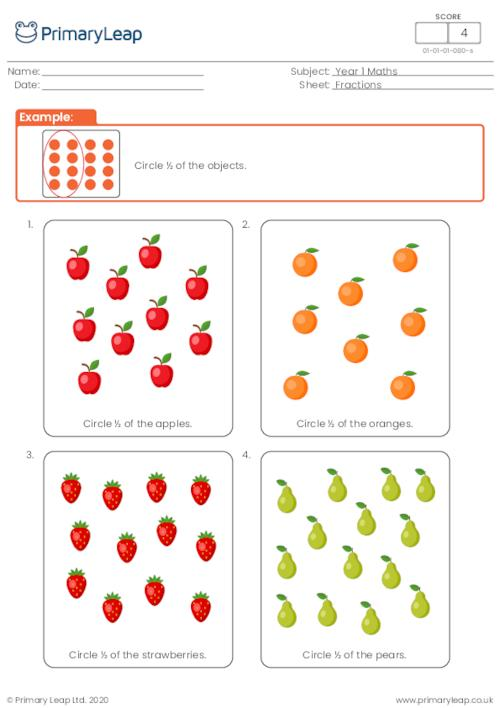### Money - Doubling the cost

Year 1 maths printable - This worksheet is all about doubling the cost. Students are asked to work out the cost of two items. There are 8 questions to answer.

Age: 5 - 6
1
2
3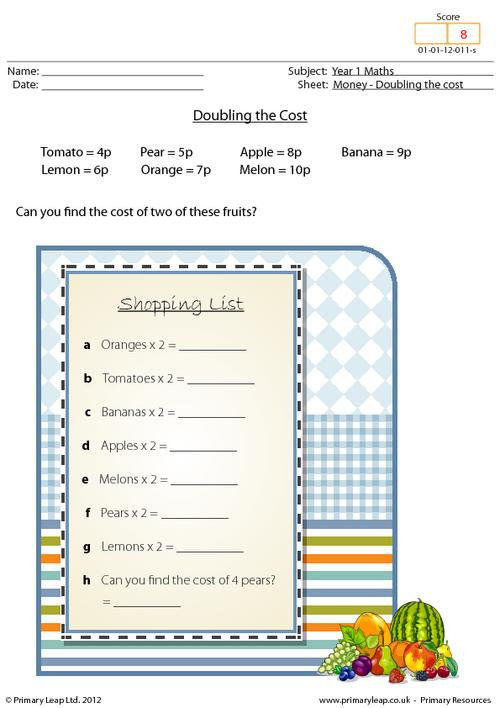### Counting in 2s

Year 1 maths printable - This worksheet practices counting in 2s. Students are firstly asked to colour in the number square correctly. They must then complete the number sequences counting in 2s.

Age: 5 - 6
1
2
3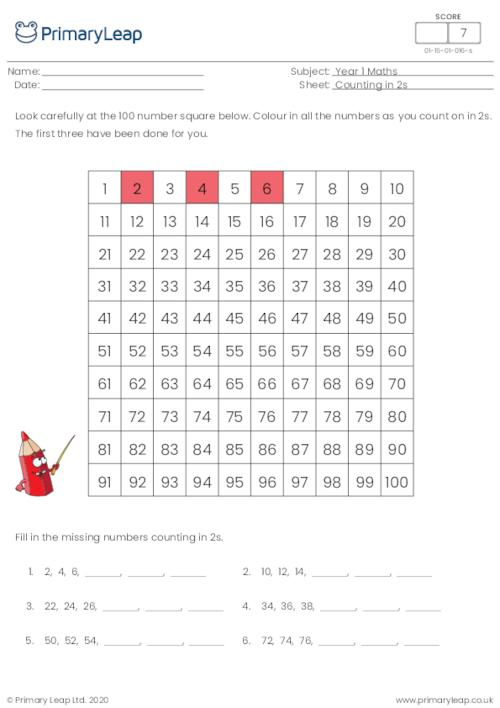### Data - Carroll diagram

We use carroll diagrams for grouping things in a yes/no fashion. Let's sort out this carroll diagram! Students have nine numbers. They must put them into the correct box in the diagram then answer the questions at the bottom of the page.

Age: 5 - 6
1
2
3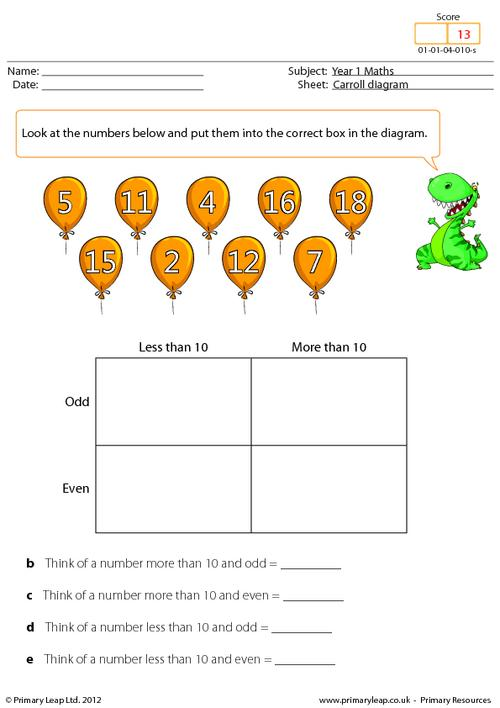### Missing numbers - 100 number square

Year 1 Maths - This page shows a 100 number square. Some of the numbers are missing. Students fill in the missing numbers to the number square and then answer the questions at the bottom of the page.

Age: 5 - 6
1
2
3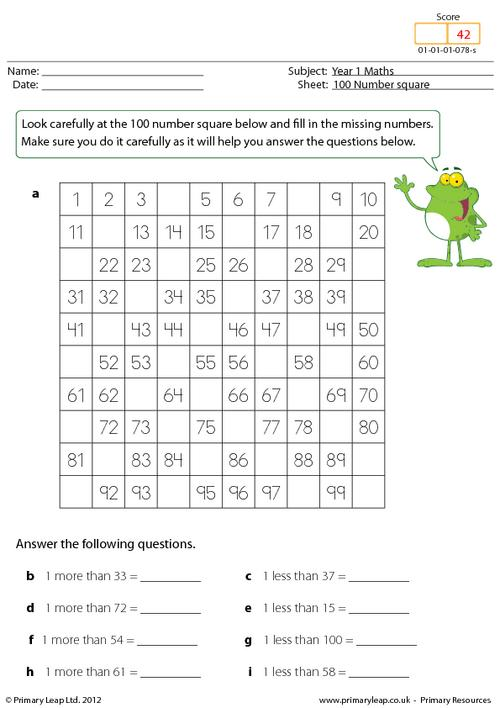### Minus 5 Spider

Year 1 Maths - This maths worksheet teaches students how to take 5 away from a given number.

Age: 5 - 6
1
2
3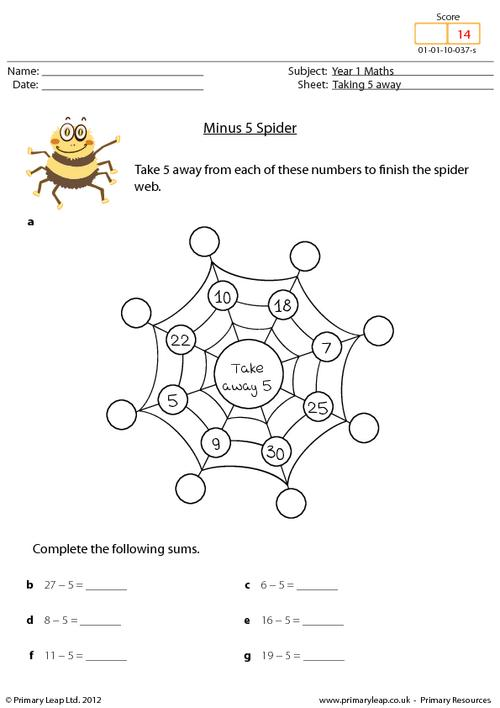Year 1 Maths - This maths worksheet teaches students how to add 5 to a given number.

Age: 5 - 6
1
2
3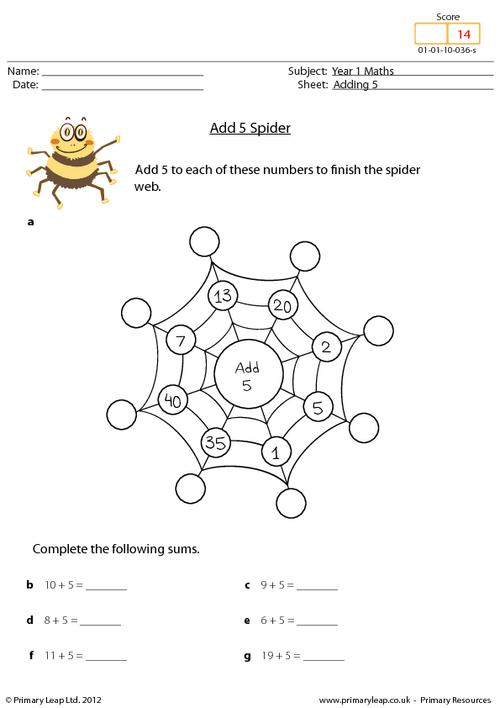Year 1 Maths - This maths worksheet teaches students how to add 10 to a given number.

Age: 5 - 6
1
2
3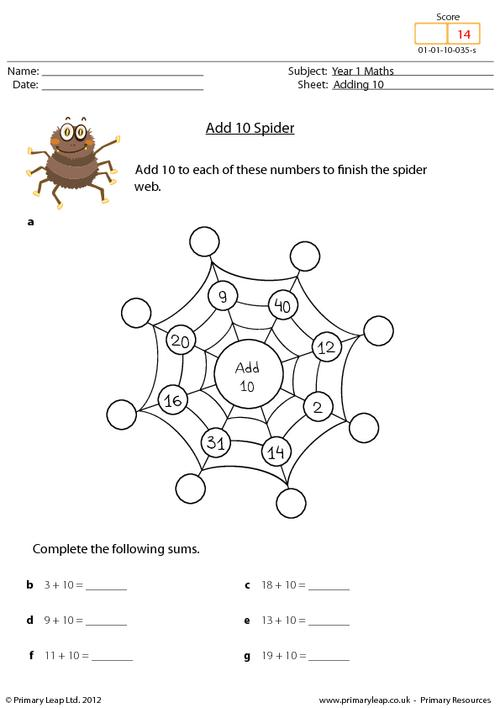### Minus 10 spider

Year 1 Maths - This maths worksheet teaches students how to take 10 away from a given number.

Age: 5 - 6
1
2
3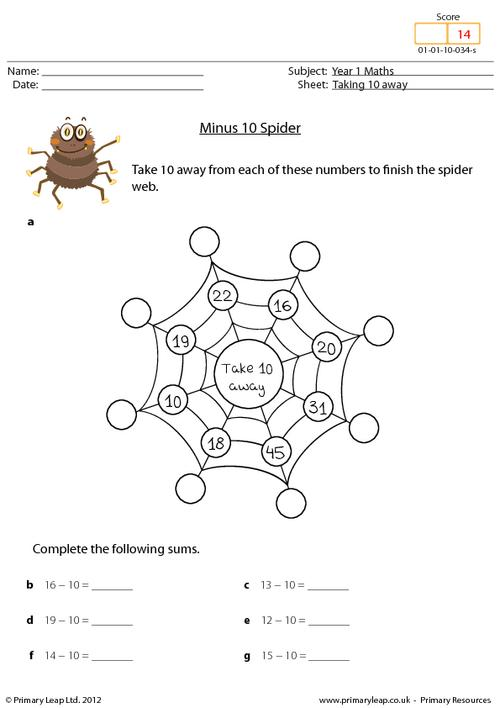### Doubling and multiplying by 2

Year 1 Maths - This maths worksheet teaches children to double and multiply by 2. Do they see a pattern?

Age: 5 - 6
1
2
3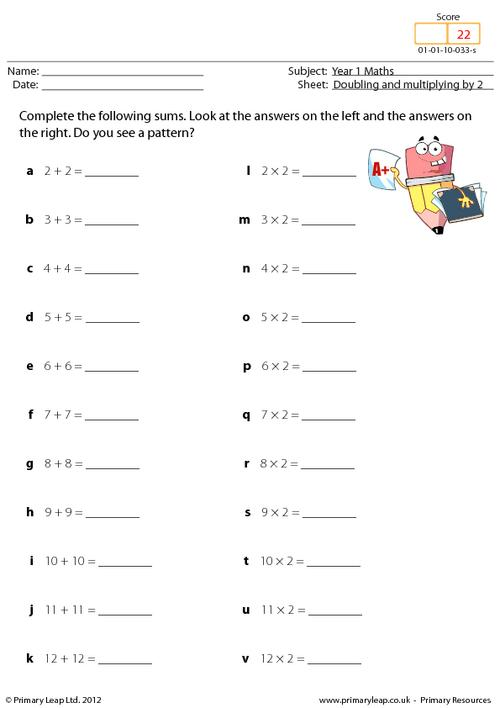### Maths investigation - Can you make 7?

Year 1 Maths - This maths worksheet asks students to think of as many ways as they can to make 7.

Age: 5 - 6
1
2
3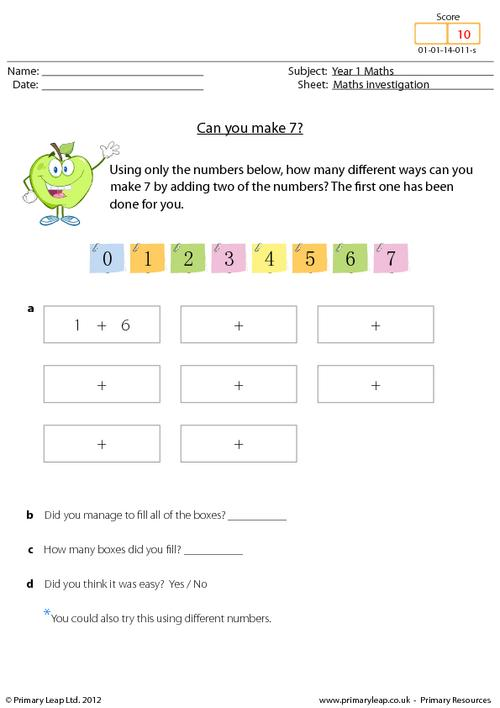### Maths investigation - Can you make 10?

Year 1 Maths - This maths worksheet asks students to think of as many ways as they can to make 10.

Age: 5 - 6
1
2
3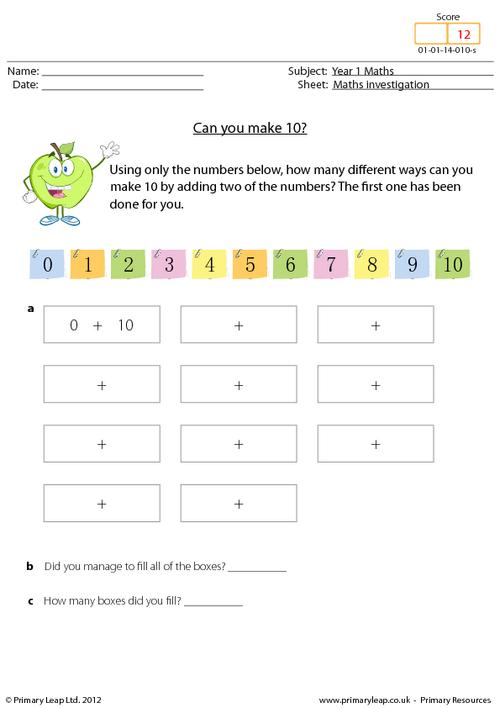### Numbers - 2 in a bag

Year 1 Maths - 2 in a bag maths worksheet. This resource asks students to put 2 of each fruit in a bag. Are there any left over?

Age: 5 - 6
1
2
3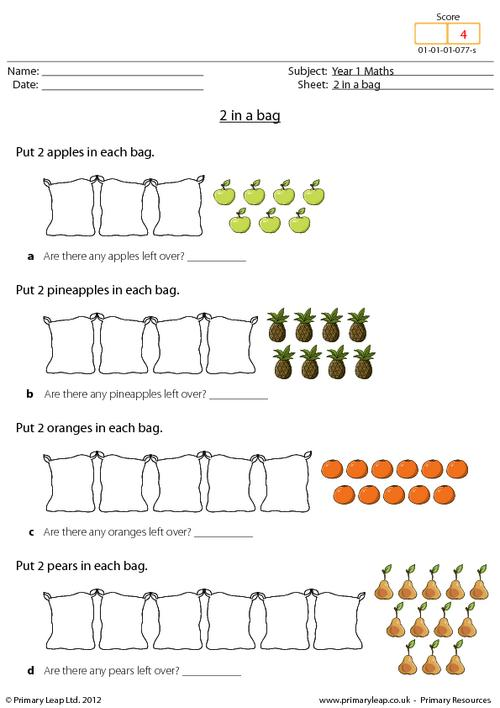### Venn diagrams - 2, 3, and 5 times table

Year 1 Maths - This worksheet teaches children how to complete a venn diagram.

Age: 5 - 6
1
2
3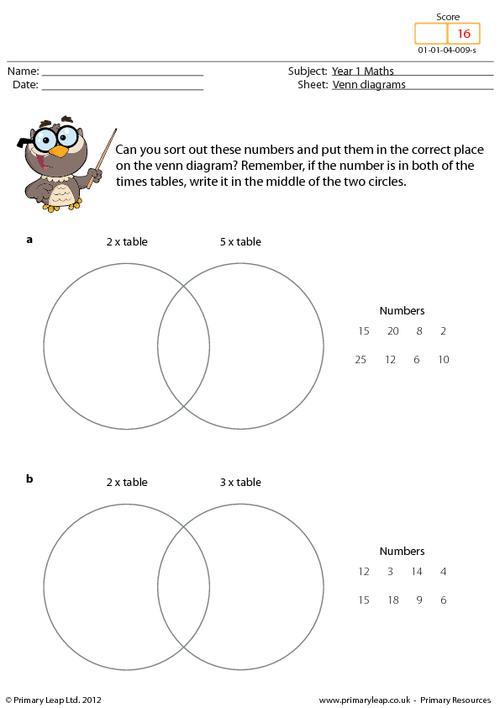### Tally charts - Pets in class 1

Year 1 Maths - Tally charts worksheet. This worksheet shows which pets the children in class 1 have. Look at the chart carefully and answer the questions.

Age: 5 - 6
1
2
3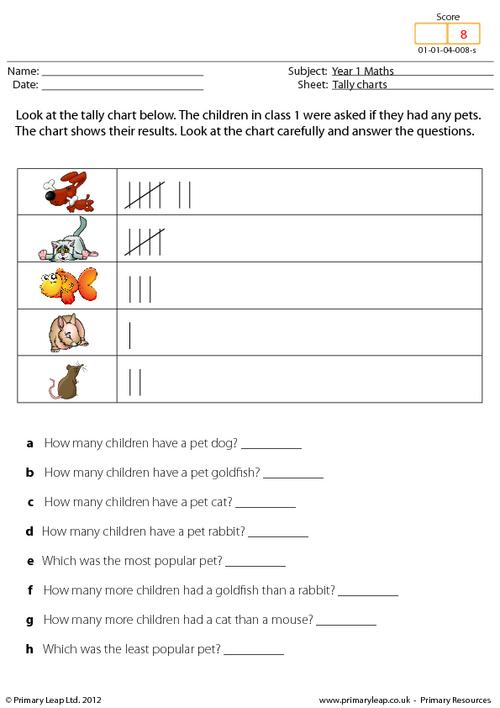### Shapes - How many cubes?

Year 1 Maths - This worksheet teaches children to count the number of cubes in each shape. They then write their answers on the lines.

Age: 5 - 6
1
2
3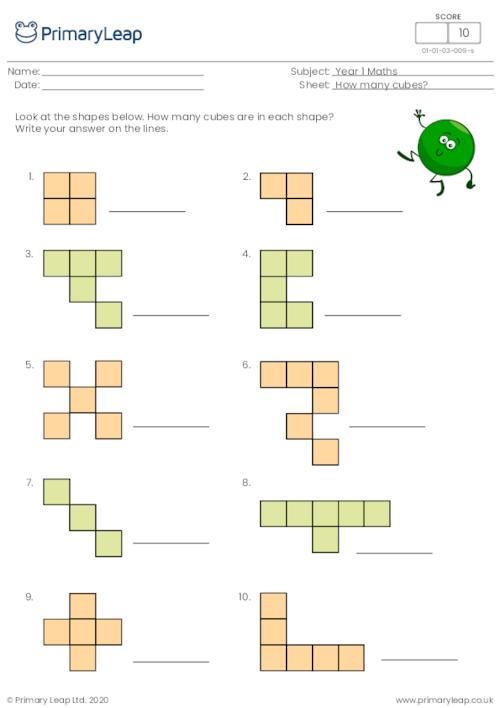### Money - Grocery shopping

Year 1 Maths - This worksheet is all about learning to add up different amounts of items and using money.

Age: 5 - 6
1
2
3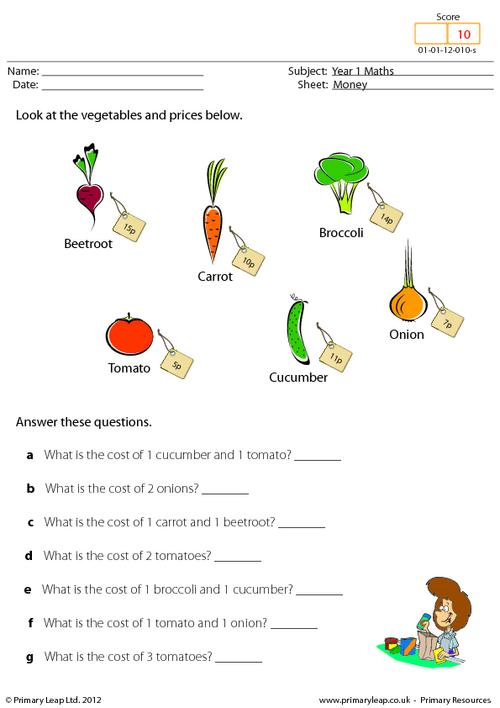### Half of an amount

Year 1 Maths - This worksheet teaches children half of an amount. Children must colour half of the shapes and then answer the division sums.

Age: 5 - 6
1
2
3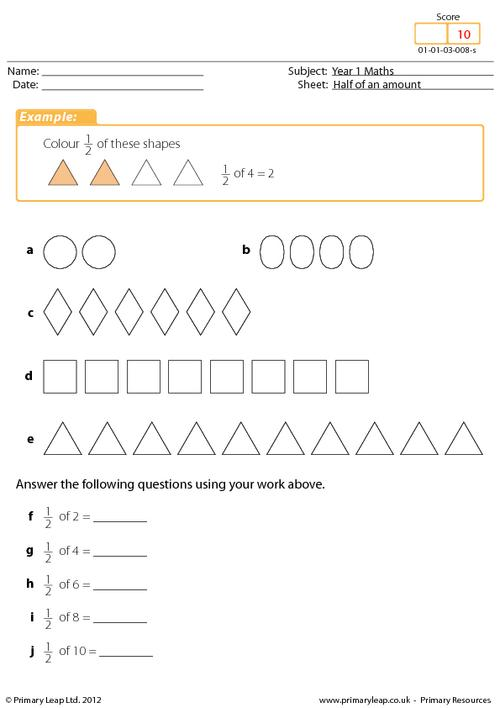### Next to, above and below

Year 1 Maths - Children must look at the balloons and answer the questions. This worksheet teaches children the terms next to, above and below.

Age: 5 - 6
1
2
3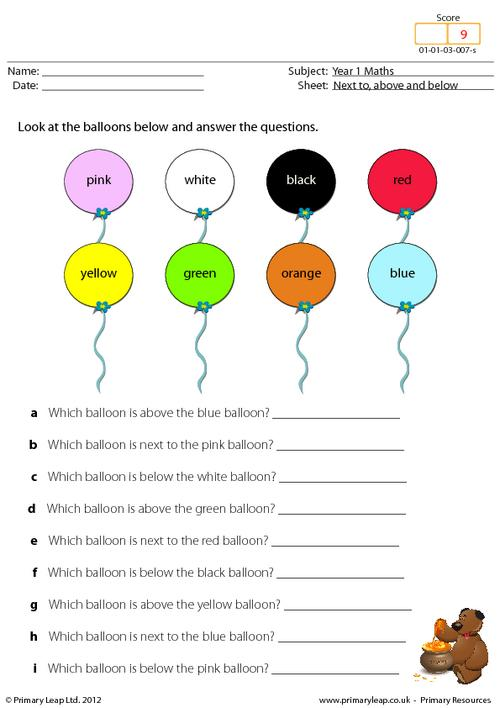### Doubling shapes

Year 1 Maths - Doubling shapes. This maths worksheet asks children to look at the shapes in the boxes and then draw double the amount in the next box.

Age: 5 - 6
1
2
3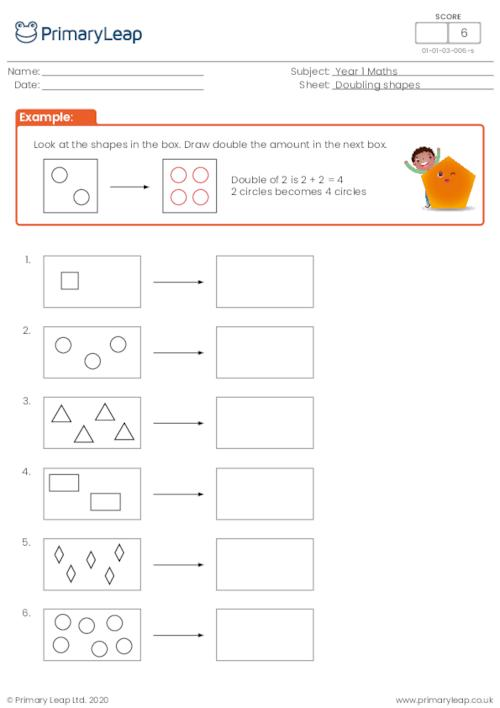### Data - How many pencils?

Year 1 Maths - This maths worksheet asks children to look at the pencils and then answer the questions.

Age: 5 - 6
1
2
3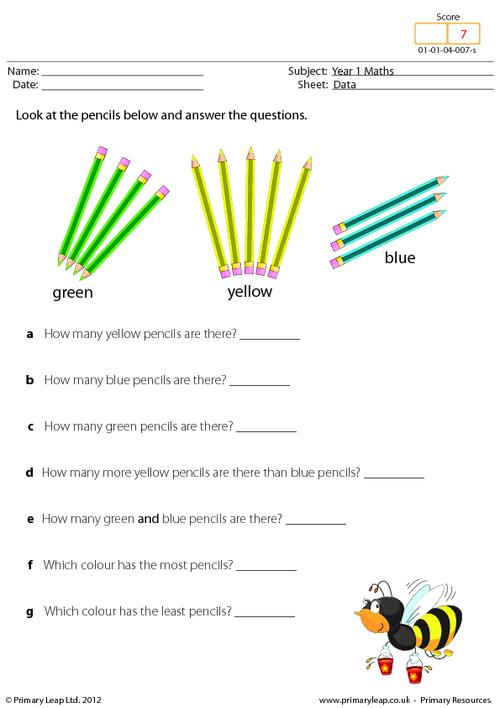### Written numbers

Year 1 Maths - This worksheet asks children to look at the numbers on the whiteboard and put them next to their correct number.

Age: 5 - 6
1
2
3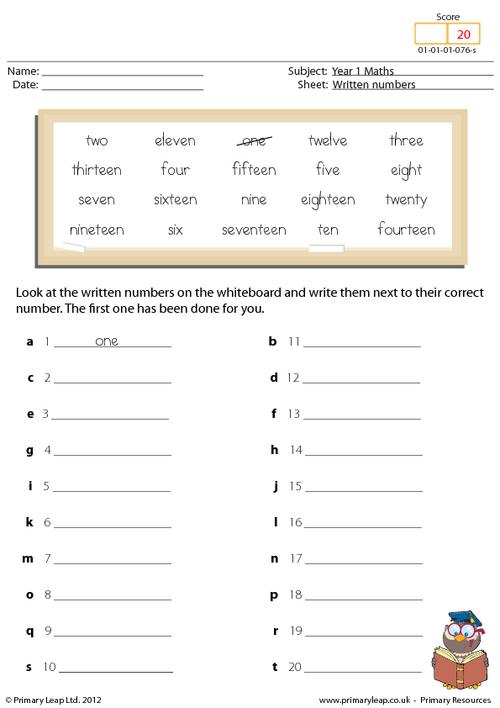### Number recognition

Year 1 Maths - Can you help Poldo the polar bear join the digit and written numbers together? The first one has been done to help you.

Age: 5 - 6
1
2
3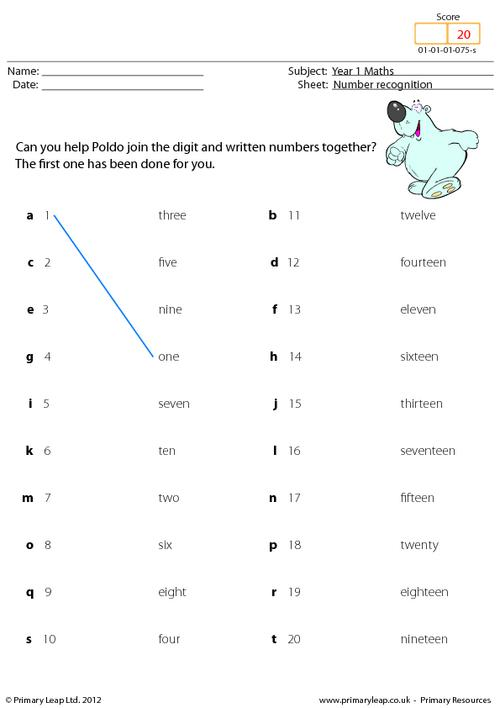### Recognising numbers

Year 1 Maths . Recognsing numbers. This maths worksheet teaches children to recognise the larger number out of a set of 5 numbers. They must colour the frog in each row with the largest number.

Age: 5 - 6
1
2
3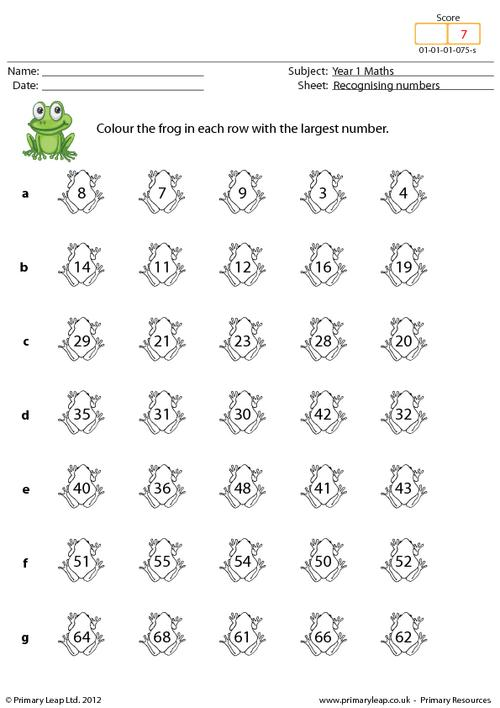### Counting in 10s

Year 1 Maths - Counting on and back in tens. This worksheet teaches children how to count forwards and backwards in 10s.

Age: 5 - 6
1
2
3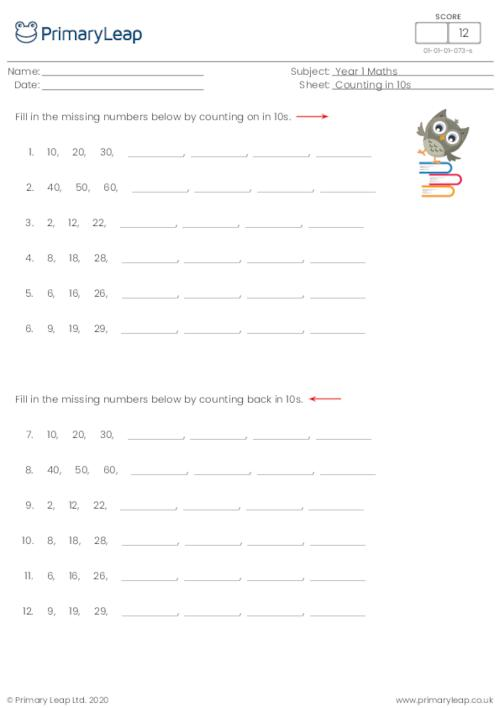### Writing numbers in words

Year 1 Maths - This worksheet teaches children how to write all of the numbers up to twenty. Some of the letters have been added to help them.

Age: 5 - 6
1
2
3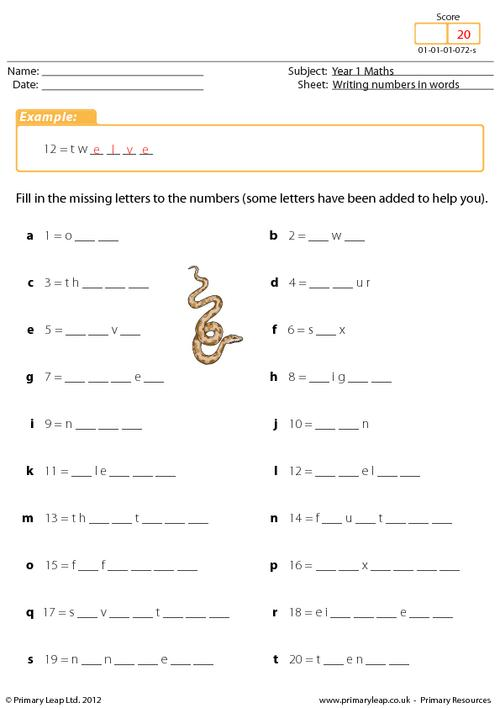### Numbers up to 100

Year 1 Maths - This maths worksheet teaches children to fill in missing numbers to 100. There are 22 questions to complete.

Age: 5 - 6
1
2
3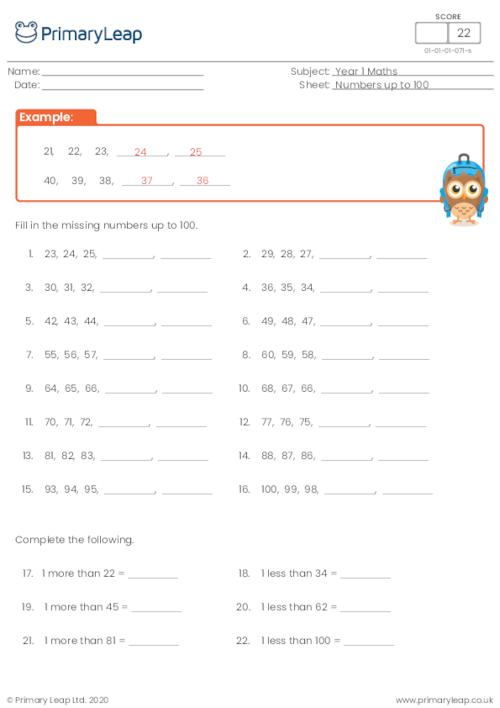### Writing numbers (3)

Year 1 Maths - This maths worksheet teaches children how to write written numbers in figures.

Age: 5 - 6
1
2
3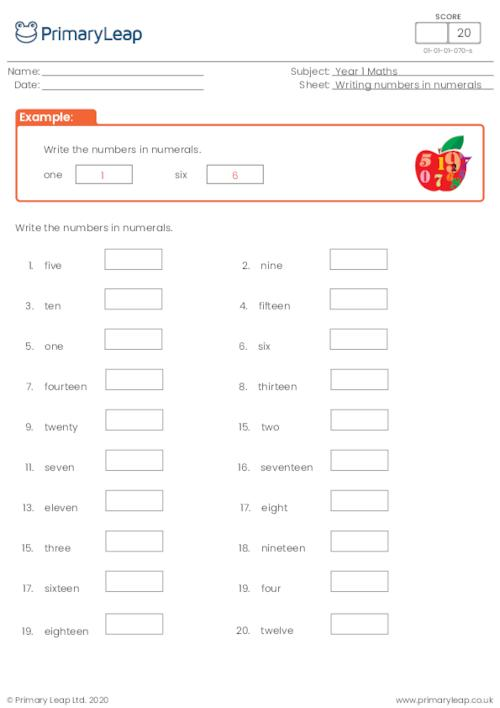### Writing numbers (2)

Year 1 Maths - This maths worksheet teaches children how to write written numbers in figures.

Age: 5 - 6
1
2
3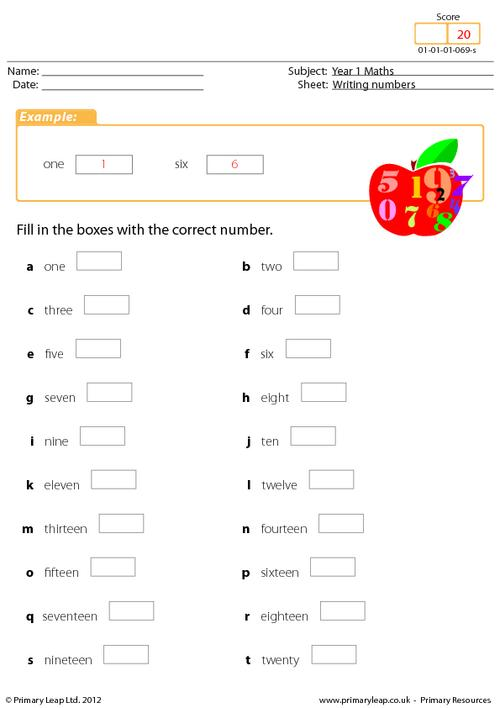### One less than

Year 1 Maths - This worksheet teaches children how to take 1 away from a given number. 1 less than and a number minus one exactly the same. There are 22 questions to answer.

Age: 5 - 6
1
2
3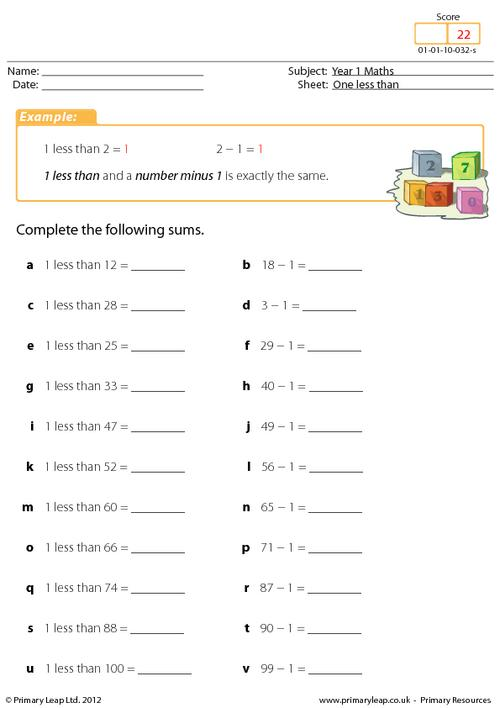of 6This is year 1 Numeracy

This is year 1 NumeracyLearnProgressImprove